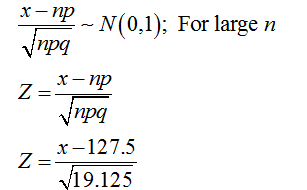# In a certain community, it is accepted that 85% of households are two-income households. You select a random sample of 150 households. What is the probability that more than 130 of them are two income households?

Question
23 views

In a certain community, it is accepted that 85% of households are two-income households. You select a random sample of 150 households. What is the probability that more than 130 of them are two income households?

check_circle

Step 1

Binomial distribution:

A random variable is said to follow binomial distribution if it satisfies the criteria given below:

• The number of observations or trails must be fixed.
• Each observation or trail must be independent.
• The probability of success must be same for all the trails.

The pmf for the binomial distribution or the probability of obtaining x successes in n independent trails of a binomial experiment is,

P(x) = nCx * px * (1 – p)n–x. Where x = 0, 1, 2, …, n and 0 < p < 1.

Here, it is given that, 85% of households are two-income households.

The probability of success (Households that are two-income) is p = 85/100 = 0.85.

The probability of failure (Households that are not two-income) is 1 – p = 0.15.

The sample number of households selected is n = 150 (Which is large in size).

The variable x be the number of households that are two income.

• Here, the number of households selected is n = 150 is fixed.
• Each of the selected 150 households are independent of each other.
• The probability of success (Households that are two-income) is p = 0.85 (Same for all 150 households).

Hence, the variable x = number of households that are two income is a binomial random variate.

Step 2

Normal approximation to binomial distribution for large sample size:

The mean and variance for the binomial variate is E(x) = n * p and V(x) = n *p *(1 – p).

Here, p = 0.85, 1 – p = 0.15 and n = 150.

Since, the sample size is large, binomial variate can be transformed to normal variate.

The mean and variance of the variable x (number of households that are two income) is E(x) = n * p = 150*0.85 = 127.5 and V(x) = n *p *(1 – p) = 150*0.85*0.15 = 19.125.

If X follows binomial distribution with parameters np and npq, the binomial variate tends to normal as follows:Step 3

Find the probability that more than 130 households are two-income:

Here, the variable number of households that are two-income is more of 130. That is, x > 130.

Th...

### Want to see the full answer?

See Solution

#### Want to see this answer and more?

Solutions are written by subject experts who are available 24/7. Questions are typically answered within 1 hour.*

See Solution
*Response times may vary by subject and question.
Tagged in

### Statistics